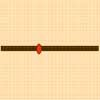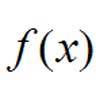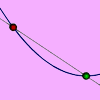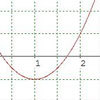# Resources tagged with: Differentiation

Filter by: Content type:
Age range:
Challenge level:

### There are 16 results

Broad Topics > Calculus > Differentiation### Impedance Can Be Complex!

##### Age 16 to 18 Challenge Level:

Put your complex numbers and calculus to the test with this impedance calculation.### Ramping it Up

##### Age 16 to 18 Challenge Level:

Look at the calculus behind the simple act of a car going over a step.### Bend

##### Age 16 to 18 Challenge Level:

What is the longest stick that can be carried horizontally along a narrow corridor and around a right-angled bend?### Integration Matcher

##### Age 16 to 18 Challenge Level:

Can you match the charts of these functions to the charts of their integrals?### Bird-brained

##### Age 16 to 18 Challenge Level:

How many eggs should a bird lay to maximise the number of chicks that will hatch? An introduction to optimisation.### Least of All

##### Age 16 to 18 Challenge Level:

A point moves on a line segment. A function depends on the position of the point. Where do you expect the point to be for a minimum of this function to occur.### Towards Maclaurin

##### Age 16 to 18 Challenge Level:

Build series for the sine and cosine functions by adding one term at a time, alternately making the approximation too big then too small but getting ever closer.### Operating Machines

##### Age 16 to 18 Challenge Level:

What functions can you make using the function machines RECIPROCAL and PRODUCT and the operator machines DIFF and INT?### Loch Ness

##### Age 16 to 18 Challenge Level:

Draw graphs of the sine and modulus functions and explain the humps.### An Introduction to Differentiation

##### Age 14 to 18

An article introducing the ideas of differentiation.### Exponential Trend

##### Age 16 to 18 Challenge Level:

Find all the turning points of y=x^{1/x} for x>0 and decide whether each is a maximum or minimum. Give a sketch of the graph.### Calculus Countdown

##### Age 16 to 18 Challenge Level:

Can you hit the target functions using a set of input functions and a little calculus and algebra?### Turning to Calculus

##### Age 16 to 18 Challenge Level:

Get started with calculus by exploring the connections between the sign of a curve and the sign of its gradient.### Slide

##### Age 16 to 18 Challenge Level:

This function involves absolute values. To find the slope on the slide use different equations to define the function in different parts of its domain.### Generally Geometric

##### Age 16 to 18 Challenge Level:

Generalise the sum of a GP by using derivatives to make the coefficients into powers of the natural numbers.### Quick Route

##### Age 16 to 18 Challenge Level:

What is the quickest route across a ploughed field when your speed around the edge is greater?